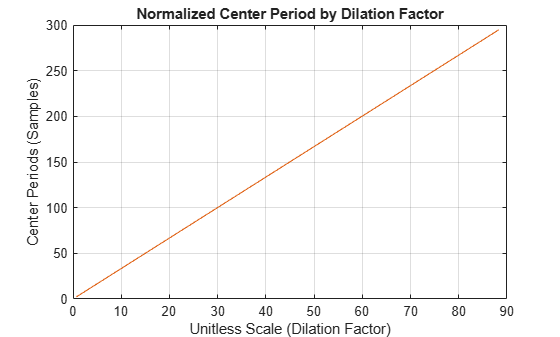# scales

CWT filter bank scales

## Syntax

``rs = scales(fb)``
``[rs,cs] = scales(fb)``

## Description

````rs = scales(fb)` returns the raw (unitless) scales used in creating the wavelet bandpass filters. Scales are ordered from finest to coarsest.```

example

````[rs,cs] = scales(fb)` returns the wavelet scales converted to units of the sampling frequency or sampling period.```

## Examples

collapse all

Create a CWT filter bank with sampling period equal to 0.001 seconds.

`fb = cwtfilterbank('SamplingPeriod',seconds(0.001));`

Obtain the raw and converted scales used in creating the wavelet bandpass filters.

`[rs,cs] = scales(fb);`

Obtain the filter bank bandpass center periods.

`P = centerPeriods(fb);`

Compare the finest converted scale with the smallest bandpass center period normalized by the sampling period.

`min(cs)`
```ans = 2.3035 ```
`min(P)/seconds(0.001)`
```ans = 2.3035 ```

The scales should increase by a factor of approximately ${2}^{1/|NV|}$, where $NV$ is the number of voices per octave. The default value of $NV$ is 10. Plot the ratios of successive scales and compare with ${2}^{1/10}$.

```len = length(rs); plot(rs(2:len)./rs(1:len-1),'rx-') hold on plot(1:len-1,2^(1/10)*ones(1,len-1),'b') title('Successive Scale Ratios') legend('Scale Ratio','Scale Factor')```## Input Arguments

collapse all

Continuous wavelet transform (CWT) filter bank, specified as a `cwtfilterbank` object.

## Output Arguments

collapse all

Raw scales used in creating the wavelet bandpass filters, returned as a real-valued vector of length Ns, where Ns is the number of wavelet bandpass frequencies (equal to the number of scales).

Data Types: `double`

Converted scales used in creating the wavelet bandpass filters, returned as a real-valued vector of length Ns, where Ns is the number of wavelet bandpass frequencies (equal to the number of scales). `cs` is in units of the sampling frequency or sampling period.

Data Types: `double`

## Version History

Introduced in R2018a